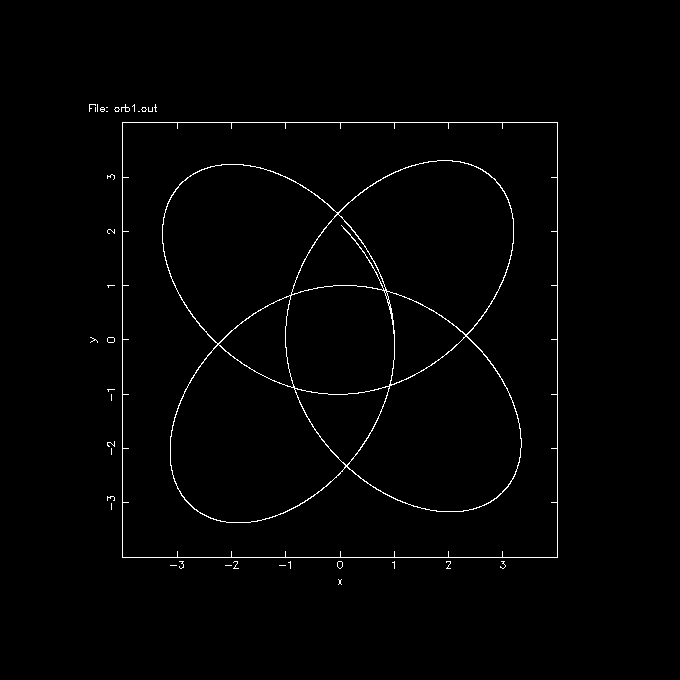# Orbits in Gridded Potentials

Here is another example how to compute orbits within NEMO, but now within a potential that is defined on a grid. This opens up an way to take real data (yes yes, from the sky).

First we need a small excursion into the obervers domain. Here are the basic steps (and you can skip steps if they've been taken already):
1. import your fits data into miriad, using fits in= out= op=xyin
2. make sure the center of the galaxy is at the reference pixel,otherwise fake it by using puthd in=map/crpix1 value= and puthd in=map/crpix2 value=. This will mess up the RA/DEC, but we only care about relative numbers here.
3. deproject the image to face on. Since the reference pixel has been set properly in the previous step, you only need to decide on the position angle and the inclination. deproject in= out= pa=xxx inc=yyy
4. use any smoothing or transformation you like to make a more realistic (?) density distribution. Perhaps even using ellint.
5. calculate the potential from the density and with an assumed scale height of the gas: potfft in= out= h=zzz. The units of scale height are the same units as the pixel, unless the distance in Mpc is given, these need to be given in pc.
6. hi saf
7. output the file in fits for importing into nemo: fits in= out= op=xyout

### NEMO

There are three ways to make an potential image that can be used with the potname=ccd descriptor:
1. Import the fits file into NEMO using fitsccd, for example from a file you exported in MIRIAD
```   fitsccd in=pot1.fits out=pot1
```
2. Use ccdmath to define an image from scratch. Here is an example of a Plummer potential:
```    ccdmath out=pot2 fie="-1/sqrt((%r/100)**2+1)" size=800,800,1 cdelt=0.01,0.01 crpix=400,400
```
Note the peculiar normalization of the radius because the pixel size is 1/100.
3. Use potccd to define an image from another potential descriptor, e.g. the same plummer potential as in the previous example:
```    potccd pot3 plummer x=-4:4:0.01 y=-4:4:0.01
```
You may want to test some values of this potential using potlist or simply display the potential in ds9 with the nds9 script.

Now create an orbit wit mkorbit

```    mkorbit orb1 x=1 y=0 z=0 vx=0 vy=1 vz=0 potname=plummer
tsf orb1
...
double IOM -0.207107 1.00000 0.00000
...
```
where for convenience the Plummer potential was also given (not required if you pass all 6 phase space coordinates) to check if the energy is bound and negative (-0.207107).

Now the orbit can be integrated using orbint using either potential descriptor:

```   orbint orb1 orb1.out 10000 0.01 potname=plummer
orbint orb1 orb2.out 10000 0.01 potname=ccd potfile=pot2
orbint orb1 orb3.out 10000 0.01 potname=ccd potfile=pot3
```
and plotted using orbplot
```   orbplot orb1.out yapp=1/xs xrange=-4:4 yrange=-4:4
orbplot orb2.out yapp=2/xs xrange=-4:4 yrange=-4:4
orbplot orb3.out yapp=3/xs xrange=-4:4 yrange=-4:4
```
and you should see they are basically all the same.### potcode

The potcode program can compute orbits in static potential with certain forms of interaction between the particles.